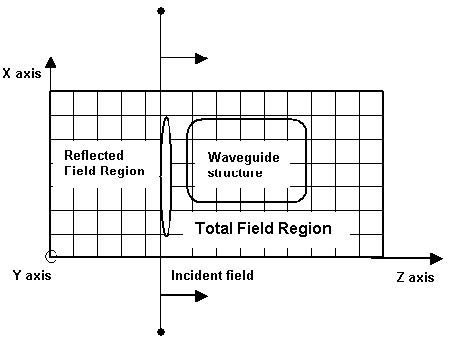The FDTD numerical scheme yields the solution of an initial value problem. At the first
time step of the simulation all the fields are set to zero. The algorithm needs the initial
field excitation that will be propagated through the computational domain. The FDTD
Total/Reflected Field formulation gives the methodology in defining a +Z propagating
incident field. The computational domain is separated into two sub-regions — the
total field region and the reflected field region. The plane separating these regions
is called the incident field (Figure 16).

In the Total Field Region, the waveguide structures of interest are designed. The
interaction between the incident field and the waveguide structure will take place in
this region. That is why the Total Field Region contains information for both the
incident and scattered (reflected) waves. In the Reflected Field Region the geometry
is uniform and the propagating waves are presented by the fields reflected from the
Total Field Region. There are no objects in this region and the signal will not be
reflected back to the total field region.Figure 16: Total/Reflected field formulation

CW or pulsed excitations can be used. One can consider the incident field as being
generated by a flashlight located on the incidence plane facing the +Z direction.

Before starting the simulation the flashlight is turned off and the field values in the
whole computational domain are equal to zero. The flashlight is switched on at t = 0
and illuminates only the Total Field Region. If the excitation scheme is perfect, there
should not be any light detected by an observer located in the Reflected Field Region,
unless there are some obstacles which would generate the reflections. The incident
wave can be generated by specifying the exact field distribution on the incident plane
at each time interval.